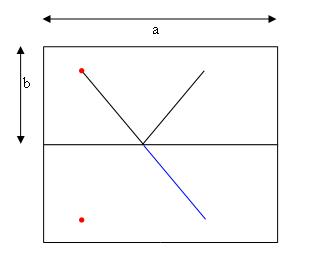#### You may also like### Coke Machine

The coke machine in college takes 50 pence pieces. It also takes a certain foreign coin of traditional design...### At a Glance

The area of a regular pentagon looks about twice as a big as the pentangle star drawn within it. Is it?### Cosines Rule

Three points A, B and C lie in this order on a line, and P is any point in the plane. Use the Cosine Rule to prove the following statement.

# Orbiting Billiard Balls

##### Age 14 to 16Challenge Level

We received solutions suggesting a number of ways to tackle this problem.

Jaime suggests a trial-and-error approach to help initially:

Drawing out the table(s) and experimenting with different paths (angles) will generate a 'feel' for the problem and begin to suggest some features and underlying relationships.

As Shaun observes,

One must assume the angle of incidence to be equal to the angle of reflection.

He goes on to suggest constructing a right angled triangle:

Let's call the length of the table $L$, the height $h$, and the angle $x$. We'll also call the total distance travelled by the ball $Z$.
Note how if we get all the straight lines that make up the ball's path, and join them end to end, we have a line of length $Z$, inclined at angle $x$ to the horizontal. Its horizontal component is $Z\cos x$, and its vertical component is $Z\sin x$.
Now, looking back at the table, we see the horizontal distance travelled is $2L$, and the vertical distance is $2h$.
So: $Z\cos x$ = $2L$
$Z\sin x$ = $2h$
$\tan x$ = $\frac{h}{L}$
$x$ = arctan $\frac{h}{L}$
The position of the ball need not be taken into account in this case.

Andrei visualised the problem by imagining that each time the ball bounced against an edge, the table was reflected:

I observe that each time a ball strikes a margin of the table it will be reflected with a reflection angle equal to the angle of incidence. Let the dimensions of the billiard table be a and b. At each collision, I reflect the whole table around its side where the ball strikes (as above), and the trajectory of the ball is a straight line.He then considered the triangle created by this straight line and the sides of the table, concluding that in the final reflection, the relative position of the red point is the same as the initial one.

In this case, $\tan x = \frac{mb}{na}$, where m and n are natural numbers

Finally, Tarj formed equations connecting time, orientation of the ball, size of the table and velocity (v1, v2). He then equated these and solved for v1/v2 = tanx. Very neatly done!